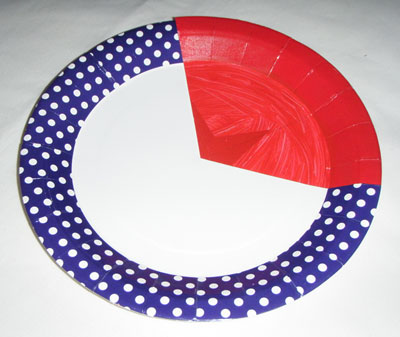Home > Fractions > Activities > Fraction wheel

# Fraction wheel

Students make a fraction wheel using two paper plates (or circles of paper or card), each a different colour or pattern. A cut is made from the edge of each plate to its centre. The plates are then slotted together and can be rotated to model fractions.Fraction wheel made from paper plates.

Watch the video How to Make a Fraction Wheel.

Specify a fraction (such as one-third) and ask students to make that fraction on their wheels and hold them up. Discuss the variations in the responses and share strategies for deciding how to position the wheel.

Make a fraction using the wheel and show it to the class very briefly. Ask pairs or small groups of students to re-create the fraction and decide how to name it. Discuss the variations in answers, and share strategies for deciding the size of the denominator and numerator for the fraction.

This task could be repeated with other commonly used shapes such as squares and rectangles, with the students making sketches of what they see when the teacher flashes the image of the fraction.